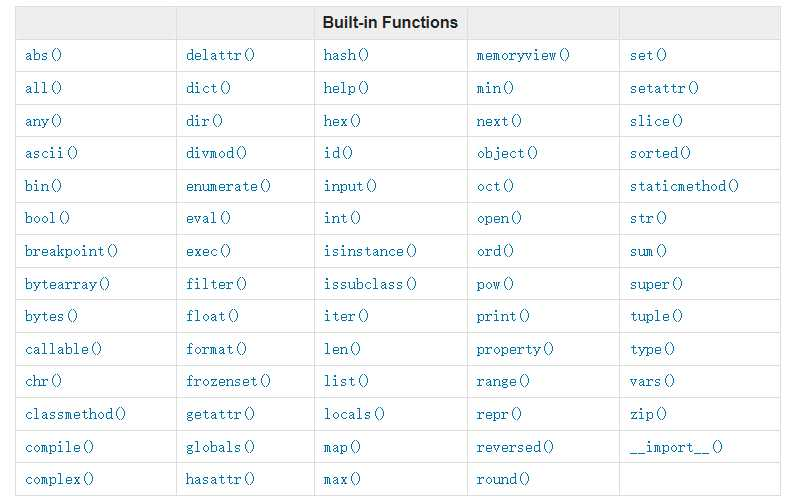# 反转列表

Python 中通常有两种反转列表的方法：切片或 reverse() 函数调用。这两种方法都可以反转列表，但需要注意的是内置函数 reverse() 会更改原始列表，而切片方法会创建一个新列表。

### 使用切片：

``````\$ python -m timeit -n 1000000 -s 'import numpy as np' 'mylist=list(np.arange(0, 200))' 'mylist[::-1]'
1000000 loops, best of 5: 15.6 usec per loop
``````

### 使用 reverse()：

``````\$ python -m timeit -n 1000000 -s 'import numpy as np' 'mylist=list(np.arange(0, 200))' 'mylist.reverse()'
1000000 loops, best of 5: 10.7 usec per loop
``````

# 交换两个值

``````variable_1 = 100
variable_2 = 500
``````

``````variable_1, variable_2 = variable_2, variable_1
``````

``````md[key_2], md[key_1] = md[key_1], md[key_2]
``````

# 在函数内部循环

``````list_of_strings = ['apple','orange','banana','pineapple','grape']
``````

``````def only_function(x):
new_string = x.capitalize()
out_putstring = x + " " + new_string
print(output_string)
``````

``````def for_in_function(listofstrings):
for x in list_of_strings:
new_string = x.capitalize()
output_string = x + " " + new_string
print(output_string)
``````

# 减少函数调用次数

``````# Check if num an int type
type(num) == type(0) # Three function calls
type(num) is type(0) # Two function calls
isinstance(num,(int)) # One function call
``````

``````# Each loop the len(a) will be called
while i < len(a):
statement
# Only execute len(a) once
m = len(a)
while i < m:
statement
``````